# Numerical solutions of 2-D unsteady incompressible flow with heat transfer in a driven square cavity using streamfunction-vorticity formulation

Numerical solutions of 2-D unsteady incompressible flow with heat transfer in a driven square cavity using streamfunction-vorticity formulation

Vusala AmbethkarManoj Kumar

Department of Mathematics, Faculty of Mathematical Sciences, University of Delhi, Delhi110007, India

Corresponding Author Email:
vambethkar@maths.du.ac.in
Page:
459-473
|
DOI:
https://doi.org/10.18280/ijht.350303
|
Accepted:
|
Published:
30 September 2017
| Citation

OPEN ACCESS

Abstract:

In this paper, we have used a streamfunction-vorticity (ψ-ξ) formulation to investigate the problem of 2-D unsteady viscous incompressible flow with heat transfer in a driven square cavity with moving top and bottom walls. We used this formulation to solve the governing equations along with no-slip and slip wall boundary conditions. A general algorithm was used for this formulation in order to compute the numerical solutions for the low Reynolds numbers Re ≤ 50. The numerical solutions of temperature are calculated for different Prandtl numbers 0.7 (for air) and 6.75 (for water). We have executed this with the aid of a computer programme developed and run in C++ compiler. We have proved the stability and convergence of the numerical scheme using matrix method. Heat transfer is studied by using the local Nusselt number. The u-velocity, v-velocity, pressure, temperature profiles along the horizontal and vertical line through geometric center of the square cavity, isotherms and isobars at different Reynolds numbers Re = 15 and 50 have been depicted.

Keywords:

components of velocity, isobars, isotherms, low and moderate reynolds numbers, no-slip and slip boundary conditions, nusselt number, stream function-vorticity formulation, two sided lid-driven square cavity

1. Introduction

The problem of 2-D unsteady viscous incompressible flow with heat transfer in a driven square cavity has wide range of applications in engineering and physical sciences. Some of these applications include oil extraction, cooling of electronic devices, heat transfer improvement in heat exchanger devices and drying technologies. The streamfunction-vorticity (ψ−ξ) formulation is a powerful approach for solving the two-dimensional viscous incompressible flow. In this method, the difficulty associated with the computation of pressure is circumvented by eliminating the pressure gradient terms from the momentum equations by cross-differentiation which leads to a vorticity-transport equation. There are some advantages as well as disadvantages associated with this method. The main advantage of this method is that it does not involve the solution of the pressure field.

Briefly discussing the related literature: Rubin and Khosla  extended the strongly implicit numerical method to the 2 ×2 coupled vorticity-streamfunction form of the Navier-Stokes equations.Ghia et al.  have used the vorticity-streamfunction formulation for the two-dimensional incompressible Naiver-Stokes equations to study the effectiveness of the coupled strongly implicit multigrid (CSI-MG) method in the determination of high-Refine-mesh flow solutions. Iliev and Makarov  have described a block-matrix iterative numerical method for coupled solving 2-D Navier-Stokes equations. Alleborn et al.  have investigated the configurations of steady two-dimensional flow accompanied by heat and mass transport in a shallow lid-driven cavity with a moving heated lid and a moving cooled lid. Natural convection in a square cavity with its horizontal walls submitted to different heating models by a finite difference procedure have been investigated by Abourida et al. . Steady state two-dimensional mixed convection problem in a vertical two sided lid-driven differentially heated square cavity was investigated numerically by Oztop and Dagtekin . Natural convection heat transfers in partially open inclined square cavities have been investigated by Bilgen and Oztop . Dhiman et al.  have investigated the flow and heat transfer characteristics of an isolated square cylinder in cross-flow placed symmetrically in a planar slit. Rahman et al.  have investigated numerical study of opposing mixed convection in a vented enclosure. The behavior of nanofluids was investigated numerically by Tiwari and Das  inside a two sided lid-driven differentially heated square cavity to gain insight into convective recirculation and flow processes induced by a nanofluid. Khanafer et al.  have investigated the numerical simulation of unsteady mixed convection in a driven cavity using an externally excited sliding lid. Luo and Yang  have studied multiple fluid flow and heat transfer solutions in a two sided lid-driven cavity. Nouanegue et al.  have investigated the conjugate heat transfer by natural convection, conduction and radiation in open cavities in which a uniform heat flux was applied to the inside surface of the solid wall facing the opening. Flow and heat transfer inside a square cavity with double-sided oscillating lids have been studied numerically by Noor et al. . Sivasankaran et al.  have investigated a numerical study on mixed convection in a lid-driven cavity with non-uniform heating on the vertical side-walls of the cavity. Mixed convection in a square cavity of sinusoidal boundary temperatures at the side walls in the presence of magnetic field was investigated numerically by Sivasankaran et al. . Wang et al.  proposed a higher order compact (HOC) finite difference solution procedure for the steady two-dimensional convection-diffusion equations in a lid-driven square cavity

filled with a CuO-water nanofluid. The mixed convection in a square porous lid-driven cavity with non-uniform heating was studied by Sivasankaran and Pan . Sivasankaran et al.  have studied a numerical analysis on mixed convection in an inclined square cavity with different sizes and locations of the heater. Steady laminar mixed convection inside a lid-driven square cavity filled with water was studied numerically by Ismael et al. . Malleswaran and Sivasankaran  have investigated on mixed convection in a lid-driven square cavity in the presence of the uniform magnetic field with corner heaters. Pandit et al.  have proposed fourth order compact scheme for the convection-diffusion equations with considering reaction and non-homogeneous source terms, to compute flow in a two sided lid-driven differentially heated square cavity filled with a fluid saturated porous medium. Chattopadhyay et al.  have numerically simulated 2-D mixed convection flow in a sinusoidally heated porous cavity whose two vertical walls (lids) are in motion.

As described in the beginning of this introduction, 2-D unsteady incompressible viscous flow with heat transfer has enormous scope of applications in engineering and physical sciences. This fact was our motivation in undertaking the current study. Moreover, the importance of these applications can be investigated only by determining numerical solutions

of the unknown flow variables, streamfunction, and vorticity function for low Reynolds numbers Re≤50. To achieve this we present, numerically, an investigation of the problem of 2-D unsteady incompressible flow with heat transfer in a driven square cavity using the streamfunction-vorticity (ψ−ξ) formulation.

The main target of this work is to suitably use streamfunction-vorticity formulation to investigate the problem of 2-D unsteady viscous incompressible flow with heat transfer in a driven square cavity with moving top and bottom walls. Alternating-Direction-Implicit (ADI) scheme has been employed to discretize the vorticity-transport equation. We have proved the stability and convergence of the numerical scheme using matrix method. A general algorithm was used for this formulation in order to compute the numerical solutions of the flow variables for Re≤50.

In this chapter, we have used a streamfunction-vorticity (ψ−ξ) formulation to investigate the problem of 2-D unsteady viscous incompressible flow with heat transfer in a driven square cavity with moving top and bottom walls. We used this formulation to solve the governing equations along with no-slip and slip wall boundary conditions. A general algorithm was used for this formulation in order to compute the numerical solutions for the flow variables: ψ, ξ, u, v, P and T for low Reynolds numbers Re≤50. The numerical solutions of the unknown flow variable T are calculated for different Prandtl numbers 0.7 (for air) and 6.75(for water). We have executed this with the aid of a computer programme developed and run in C++ compiler. We have proved the stability and convergence of the numerical scheme using matrix method. Following this, the stability conditions obtained for the time and space steps have been used in numerical computations to arrive at the numerical solutions with desired accuracy.

The design of the current work is as follows: Section 2 provides the physical description, governing equations of the 2-D unsteady incompressible viscous flow with heat transfer in a driven cavity along with the initial and boundary conditions for a square cavity, streamfunction-vorticity method and determination of pressure for viscous flow. Section 3 describes numerical discretization of governing equations and specification of boundary conditions. Section 4 provides proof of the stability and convergence of the numerical scheme using the matrix method. Section 5 provides numerical computations and general algorithm for computation of numerical solutions of the flow variables. Section 6 discusses the numerical results. Section 8 lays out the conclusions of the study.

2. Problemformulation

2.1 Physical description

Figure 1 illustrates the geometry of the problem in this study along with the boundary conditions. ABCD is a square cavity in which a 2-D unsteady incompressible viscous flow with heat transfer is considered. Now, by sliding in finite long plates lying on top and bottom of the cavity, vorticity along the walls of the cavity is generated. Suppose that all variables are normalized so that the size of the cavity is 1×1 and the sliding velocities are 1 and -1 in the positive and negative x-directions respectively. The temperature on the walls AB and DC are T=-0.5 and T=0.5 respectively.

## 1.png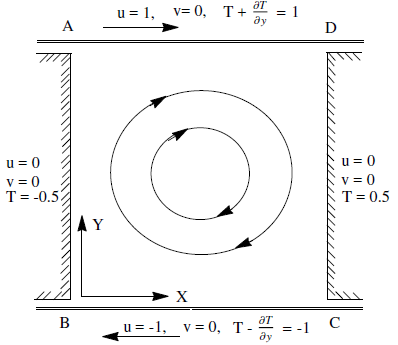Figure 1. Square cavity flow caused by moving plates with temperature boundary conditions

The boundary conditions are defined as no slip on the stationary walls (AB and DC) and as slip on the moving walls (AD and BC). We have assumed that, at all the four corner points of the square domain, the velocity components (u, v), temperature T and pressure P vanish.

2.2 Governing equations

In the present investigation, unsteady 2-D incompressible flow with heat transfer in a driven square cavity with no-slip and slip wall boundary conditions has been considered. The governing equations of 2-D unsteady incompressible viscous flow with heat transfer are the continuity equation, and the two components of the momentum equations and the energy equation. Using the Boussinesq approximations, the non-dimensional governing equations of this problem are expressed as follows:

$\frac{\partial u}{\partial x}+\frac{\partial v}{\partial y}=0$     (1)

$\frac{\partial u}{\partial t}+u \frac{\partial u}{\partial x}+v \frac{\partial u}{\partial y}=-\frac{\partial P}{\partial x}+\frac{1}{\operatorname{Re}}\left(\frac{\partial^{2} u}{\partial x^{2}}+\frac{\partial^{2} u}{\partial y^{2}}\right)$    (2)

$\frac{\partial v}{\partial t}+u \frac{\partial v}{\partial x}+v \frac{\partial v}{\partial y}=-\frac{\partial P}{\partial y}+\frac{1}{\operatorname{Re}}\left(\frac{\partial^{2} v}{\partial x^{2}}+\frac{\partial^{2} v}{\partial y^{2}}\right)$       (3)

$\frac{\partial T}{\partial t}+u \frac{\partial T}{\partial x}+v \frac{\partial T}{\partial y}=\frac{1}{\operatorname{Pr}}\left(\frac{\partial^{2} T}{\partial x^{2}}+\frac{\partial^{2} T}{\partial y^{2}}\right)$     (4)

where u, v, P, T, Re, and Pr are the velocity components along x and y axis, pressure, temperature, Reynolds number and Prandtl number respectively. The initial, no-slip and slip wall boundary conditions are

For t=0,

$u(x, y, 0)=0, v(x, y, 0)=0, T(x, y, 0)=0$

For $t>0$ ,

on plate AB:  $u=0, \quad v=0, \quad T=-0.5$

on plate DC:  $u=0, \quad v=0, \quad T=0.5$

on plate AD:  $u=1, \quad v=0, \quad T+\frac{\partial T}{\partial y}=1$

on plate BC:  $u=-1, \quad v=0, \quad T-\frac{\partial T}{\partial y}=-1$

2.3 Streamfunction-vorticity formulation

To obtain the streamfunction-vorticity (ψ−ξ) equation, pressure $P$ is eliminated from Eq. (2) and Eq. (3) by differentiating Eq. (2) with respect to $y$ and Eq. (3) with respect to $x$ and subtracting one from the other. The resulting equation is expressed with vorticity ξ as the dependent variable which is defined by

$\xi=\frac{\partial v}{\partial x}-\frac{\partial u}{\partial y}$   (5)

The result is

$\frac{\partial \xi}{\partial t}+u \frac{\partial \xi}{\partial x}+v \frac{\partial \xi}{\partial y}=\frac{1}{\operatorname{Re}}\left(\frac{\partial^{2} \xi}{\partial x^{2}}+\frac{\partial^{2} \xi}{\partial y^{2}}\right)$   (6)

Equation (6) is a parabolic PDE called vorticity-transport equation expressed in compact form as follows:

$\frac{D \xi}{D t}=\frac{1}{R e} \nabla^{2} \xi$   (7)

Now, define a streamfunction $\psi$ as

$\frac{\partial \psi}{\partial x}=-v, \frac{\partial \psi}{\partial y}=u$   (8)

$\psi$ Identically, satisfies the continuity Eq. (1). Further, use of the definition of ξ leads to

$\frac{\partial^{2} \psi}{\partial x^{2}}+\frac{\partial^{2} \psi}{\partial y^{2}}=-\xi$   (9)

This is an elliptic PDE called Poisson equation. The energy Eq. (4) can be expressed as

$\frac{\partial T}{\partial t}=\frac{1}{\operatorname{Pr}}\left(\frac{\partial^{2} T}{\partial x^{2}}+\frac{\partial^{2} T}{\partial y^{2}}\right)+\left(\frac{\partial \psi}{\partial x}\right)\left(\frac{\partial T}{\partial y}\right)-\left(\frac{\partial \psi}{\partial y}\right)\left(\frac{\partial T}{\partial x}\right)$   (10)

2.4 Determination of pressure for viscous flow

In the streamfunction-vorticity method, to obtain pressure at each grid point for viscous flow, it is necessary to solve an additional equation for pressure. This equation is derived by differentiating Eq. (2) with respect to x and Eq. (3) with respect to y and adding them together. The resulting equation is expressed as follows:

$\frac{\partial}{\partial t}\left(\frac{\partial u}{\partial x}+\frac{\partial v}{\partial y}\right)+\left[\left(\frac{\partial u}{\partial x}+\frac{\partial v}{\partial y}\right)^{2}-2\left(\frac{\partial u}{\partial x}\right)\left(\frac{\partial v}{\partial y}\right)\right]+2\left(\frac{\partial v}{\partial x}\right)\left(\frac{\partial u}{\partial y}\right)$ $+u\left\{\frac{\partial}{\partial x}\left(\frac{\partial u}{\partial x}+\frac{\partial v}{\partial y}\right)\right\}+v\left\{\frac{\partial}{\partial y}\left(\frac{\partial u}{\partial x}+\frac{\partial v}{\partial y}\right)\right\}$ $=-\nabla^{2} P+\frac{1}{R e}\left[\frac{\partial^{2}}{\partial x^{2}}\left(\frac{\partial u}{\partial x}+\frac{\partial v}{\partial y}\right)+\frac{\partial^{2}}{\partial y^{2}}\left(\frac{\partial u}{\partial x}+\frac{\partial v}{\partial y}\right)\right]$  (11)

From the continuity equation for incompressible flow, we have

$\frac{\partial u}{\partial x}+\frac{\partial v}{\partial y}=0$

We obtain from Eq. (11),

$\nabla^{2} P=2\left[\left(\frac{\partial u}{\partial x}\right)\left(\frac{\partial v}{\partial y}\right)-\left(\frac{\partial v}{\partial x}\right)\left(\frac{\partial u}{\partial y}\right)\right]$ or  $\nabla^{2} P=S$ (12)

Eq. (12) is known as Poisson equation for pressure. A suitable second-order difference representation for right hand side of Eq. (12) is given as

$S_{i, j}=2\left[\left(\frac{\psi_{i+1, j}-2 \psi_{i, j}+\psi_{i-1, j}}{\Delta x^{2}}\right)\left(\frac{\psi_{i, j+1}-2 \psi_{i, j}+\psi_{i, j-1}}{\Delta y^{2}}\right)\right.$  $-\left(\frac{\psi_{i+1, j+1}-\psi_{i+1, j-1}-\psi_{i-1, j+1}+\psi_{i-1, j-1}}{4 \Delta x \Delta y}\right)^{2} ]$      (13)

3. Numerical Discretization

## 2.png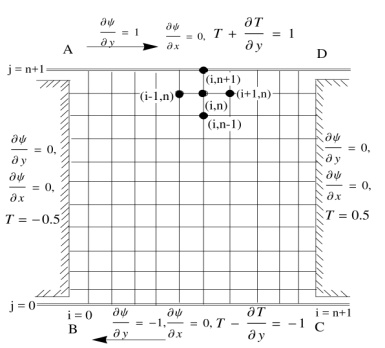Figure 2. Finite difference grid of a square cavity flow caused by moving plates with temperature boundary conditions

Discretization of the governing equations by finite difference method although a well-known technique we have adopted this technique in the present study due to its compatibility with the regularly shaped geometry, flow in a square cavity caused by moving plates. With the help of streamfunction-vorticity (ψ-ξ) formulation as discussed above, the governing equations (1) to (4) of 2-D unsteady incompressible viscous flow with heat transfer in non-dimensional form are expressed as:

$\frac{\partial^{2} \psi}{\partial x^{2}}+\frac{\partial^{2} \psi}{\partial y^{2}}=-\xi$   (14)

$\frac{\partial \xi}{\partial t}=\frac{1}{R e}\left(\frac{\partial^{2} \xi}{\partial x^{2}}+\frac{\partial^{2} \xi}{\partial y^{2}}\right)+\left(\frac{\partial \psi}{\partial x}\right)\left(\frac{\partial \xi}{\partial y}\right)-\left(\frac{\partial \psi}{\partial y}\right)\left(\frac{\partial \xi}{\partial x}\right)$   (15)

$\frac{\partial T}{\partial t}=\frac{1}{P r}\left(\frac{\partial^{2} T}{\partial x^{2}}+\frac{\partial^{2} T}{\partial y^{2}}\right)+\left(\frac{\partial \psi}{\partial x}\right)\left(\frac{\partial T}{\partial y}\right)-\left(\frac{\partial \psi}{\partial y}\right)\left(\frac{\partial T}{\partial x}\right)$   (16)

where Re is the Reynolds number, Pr is the Prandtl number, x and y are the Cartesian co-ordinates. Essentially, the system is composed of the Poisson equation for streamfunction Eq. (14), the vorticity-transport equation Eq. (15) and the energy equation Eq. (16).

To obtain the numerical solutions, the coupled equations (14) to (16) need to be solved in an iterative manner. There is a distinct advantage in using a scheme that will allow equations (15) and (16) to be solved by means of tridiagonal forms only. Therefore, a fully implicit scheme, namely Alternating-Direction-Implicit (ADI) scheme is used for solving numerically these coupled partial differential equations (15) and (16).

Consider a square numerical grid of size 1×1 having n horizontal interior grid lines and an equal number of vertical grid lines as shown in Figure 2. Using the ADI scheme [5, pp.883] and applying the forward-time and central-space (FTCS) finite difference quotients for the first and second order partial derivatives of $\psi$ , ξ and T with respect to time and space variables in equations (14) to (16) as given below:

$\frac{\partial^{2} \xi}{\partial x^{2}}\left|t=\frac{\xi_{i+1, j}^{t}-2 \xi_{i, j}^{t}+\xi_{i-1, j}^{t}}{\Delta x^{2}}, \frac{\partial^{2} T}{\partial x^{2}}\right|_{t}=\frac{T_{i+1, j}^{t}-2 T_{i, j}^{t}+T_{i-1, j}^{t}}{\Delta x^{2}}$

$\left.\frac{\partial^{2} \xi}{\partial y^{2}}\right|_{t+\frac{1}{2}}=\frac{\xi_{i, j+1}^{t+\frac{1}{2}}-2 \xi_{i, j}^{t+\frac{1}{2}}+\xi_{i, j-1}^{t+\frac{1}{2}}}{\Delta y^{2}},\left.\frac{\partial^{2} T}{\partial y^{2}}\right|_{t+\frac{1}{2}}=\frac{T_{i, j+1}^{t+\frac{1}{2}}-2 T_{i, j}^{t+\frac{1}{2}}+T_{i, j-1}^{t+\frac{1}{2}}}{\Delta y^{2}}$

$\left.\frac{\partial \xi}{\partial x}\right|_{t}=\frac{\xi_{i+1, j}^{t}-\xi_{i-1, j}^{t}}{2 \Delta x},\left.\frac{\partial T}{\partial x}\right|_{t}=\frac{T_{i+1, j}^{t}-T_{i-1, j}^{t}}{2 \Delta x}$

$\left.\frac{\partial \xi}{\partial y}\right|_{t+\frac{1}{2}}=\frac{\xi_{i, j+1}^{t+\frac{1}{2}}-\xi_{i, j-1}^{t+\frac{1}{2}}}{2 \Delta y},\left.\frac{\partial T}{\partial y}\right|_{t+\frac{1}{2}}=\frac{T_{i, j+1}^{t+\frac{1}{2}}-T_{i, j-1}^{t+\frac{1}{2}}}{2 \Delta y}$

$\left.\frac{\partial \xi}{\partial t}\right|_{t}=\frac{\xi_{i, j}^{t+\frac{1}{2}}-\xi_{i, j}^{t}}{\frac{\Delta t}{2}},\left.\frac{\partial T}{\partial t}\right|_{t}=\frac{T_{i, j}^{t+\frac{1}{2}}-T_{i, j}^{t}}{\frac{\Delta t}{2}}$

$\left.\frac{\partial \psi}{\partial x}\right|_{t}=\frac{\psi_{i+1, j}^{t}-\psi_{i-1, j}^{t}}{2 \Delta x},\left.\frac{\partial \psi}{\partial y}\right|_{t}=\frac{\psi_{i, j+1}^{t}-\psi_{i, j-1}^{t}}{2 \Delta y}$

$\left.\frac{\partial^{2} \psi}{\partial x^{2}}\right|_{t}=\frac{\psi_{i+1, j}^{t}-2 \psi_{i, j}^{t}+\psi_{i-1, j}^{t}}{\Delta x^{2}},\left.\frac{\partial^{2} \psi}{\partial y^{2}}\right|_{t}=\frac{\psi_{i, j+1}^{t}-2 \psi_{i, j}^{t}+\psi_{i, j-1}^{t}}{\Delta y^{2}}$

The discretized Poisson Eq. (14) for $\psi$ can be written as

$\frac{\psi_{i+1, j}^{t+1}-2 \psi_{i, j}^{t+1}+\psi_{i-1, j}^{t+1}}{\Delta x^{2}}+\frac{\psi_{i, j+1}^{t+1}-2 \psi_{i, j}^{t+1}+\psi_{i, j-1}^{t+1}}{\Delta y^{2}}=-\xi_{i, j}^{t+1}$

We choose $\Delta \mathrm{x}=\Delta \mathrm{y}$ so that the above discretized equation reduces to

$\psi_{i+1, j}^{t+1}+\psi_{i-1, j}^{t+1}+\psi_{i, j+1}^{t+1}+\psi_{i, j-1}^{t+1}-4 \psi_{i, j}^{t+1}=-\xi_{i, j}^{t+1} \Delta x^{2}$   (17)

The discretized vorticity-transport Eq. (15) over time step t+1/2 can be written as

$\frac{\xi_{i, j}^{t+\frac{1}{2}}-\xi_{i, j}^{t}}{\Delta t}=\frac{1}{2 R e}\left(\frac{\xi_{i+1, j}^{t}-2 \xi_{i, j}^{t}+\xi_{i-1, j}^{t}}{\Delta x^{2}}\right)-\frac{1}{2}\left(\frac{\partial \psi}{\partial y}\right)^{t}\left[\frac{\xi_{i+1, j}^{t}-\xi_{i-1, j}^{t}}{2 \Delta x}\right]$  $+\frac{1}{2}\left(\frac{\partial \psi}{\partial x}\right)^{t}\left[\frac{\xi_{i, j+1}^{t+\frac{1}{2}}-\xi_{i, j-1}^{t+\frac{1}{2}}}{2 \Delta y}\right]+\frac{1}{2 R e}\left[\frac{\xi_{i, j+1}^{t+\frac{1}{2}}-2 \xi_{i, j}^{t+\frac{1}{2}}+\xi_{i, j-1}^{t+\frac{1}{2}}}{\Delta y^{2}}\right]$

Rearranging terms in the above equation, we have

$\left[\frac{\Delta t}{4 \Delta y}\left(\frac{\psi_{i+1, j}^{t}-\psi_{i-1, j}^{t}}{2 \Delta x}\right)-\frac{\Delta t}{2 \operatorname{Re} \Delta y^{2}}\right] \xi_{i, j-1}^{t+\frac{1}{2}}+\left(1+\frac{\Delta t}{\operatorname{Re} \Delta y^{2}}\right) \xi_{i, j}^{t+\frac{1}{2}}$ $+\left[-\frac{\Delta t}{4 \Delta y}\left(\frac{\psi_{i+1, j}^{t}-\psi_{i-1, j}^{t}}{2 \Delta x}\right)-\frac{\Delta t}{2 R e \Delta y^{2}}\right] \xi_{i, j+1}^{t+\frac{1}{2}}=\xi_{i, j}^{t}+\frac{\Delta t}{2 \operatorname{Re} \Delta x^{2}}$ $\times\left[\xi_{i+1, j}^{t}-2 \xi_{i, j}^{t}+\xi_{i-1, j}^{t}\right]-\frac{\Delta t}{8 \Delta r \Delta y}\left[\xi_{i+1, j}^{t}-\xi_{i-1, j}^{t}\right]\left[\psi_{i, j+1}^{t}-\psi_{i, j-1}^{t}\right]$

This is the final discretized equation of Eq. (15) at time step t+1/2. Now, discretizing the energy equation Eq. (16) over time step t+1/2 using the ADI scheme we have

$\frac{T_{i, j}^{t+\frac{1}{2}}-T_{i, j}^{t}}{\Delta t}=\frac{1}{2 P r}\left(\frac{T_{i+1, j}^{t}-2 T_{i, j}^{t}+T_{i-1, j}^{t}}{\Delta x^{2}}\right)-\frac{1}{2}\left(\frac{\partial \psi}{\partial y}\right)^{t}\left[\frac{T_{i+1, j}^{t}-T_{i-1, j}^{t}}{2 \Delta x}\right]$ $+\frac{1}{2}\left(\frac{\partial \psi}{\partial x}\right)^{t}\left[\frac{T_{i, j+1}^{t+\frac{1}{2}}-T_{i, j-1}^{t+\frac{1}{2}}}{2 \Delta y}\right]+\frac{1}{2 P r}\left[\frac{T_{i, j+1}^{t+\frac{1}{2}}-2 T_{i, j}^{t+\frac{1}{2}}+T_{i, j-1}^{t+\frac{1}{2}}}{\Delta y^{2}}\right]$

Rearranging terms in the preceding equation, we have

$\left[\frac{\Delta t}{4 \Delta y}\left(\frac{\psi_{i+1, j}^{t}-\psi_{i-1, j}^{t}}{2 \Delta x}\right)-\frac{\Delta t}{2 \operatorname{Pr} \Delta y^{2}}\right] T_{i, j-1}^{t+\frac{1}{2}}+\left(1+\frac{\Delta t}{\operatorname{Pr} \Delta y^{2}}\right) T_{i, j}^{t+\frac{1}{2}}$ $+\left[-\frac{\Delta t}{4 \Delta y}\left(\frac{\psi_{i+1, j}^{t}-\psi_{i-1, j}^{t}}{2 \Delta x}\right)-\frac{\Delta t}{2 P r \Delta y^{2}}\right] T_{i, j+1}^{t+\frac{1}{2}}=T_{i, j}^{t}+\frac{\Delta t}{2 P r \Delta x^{2}}$ $\times\left[T_{i+1, j}^{t}-2 T_{i, j}^{t}+T_{i-1, j}^{t}\right]-\frac{\Delta t}{8 \Delta x \Delta y}\left[T_{i+1, j}^{t}-T_{i-1, j}^{t}\right]\left[\psi_{i, j+1}^{t}-\psi_{i, j-1}^{t}\right]$    (18)

This is the final discretized equation of Eq. (16) at time step t+1/2. Now, discretizing the vorticity-transport equation Eq. (15) and the energy equation Eq. (16) over time step t+1 by introducing the finite difference quotients as described below:

$\left.\frac{\partial^{2} \xi}{\partial x^{2}}\right|_{t+1}=\frac{\xi_{i+1, j}^{t+1}-2 \xi_{i, j}^{t+1}+\xi_{i-1, j}^{t+1}}{\Delta x^{2}},\left.\frac{\partial^{t+1}}{\partial x^{2}}\right|_{t+1}=\frac{T_{i+1, j}^{t+1}-2 T_{i, j}^{t+1}+T_{i-1, j}^{t+1}}{\Delta x^{2}}$

$\left.\frac{\partial^{2} \xi}{\partial y^{2}}\right|_{t+\frac{1}{2}}=\frac{\xi_{i, j+1}^{t+\frac{1}{2}}-2 \xi_{i, j}^{t+\frac{1}{2}}+\xi_{i, j-1}^{t+\frac{1}{2}}}{\Delta y^{2}},\left.\frac{\partial^{2} T}{\partial y^{2}}\right|_{t+\frac{1}{2}}=\frac{T_{i, j+1}^{t+\frac{1}{2}}-2 T_{i, j}^{t+\frac{1}{2}}+T_{i, j-1}^{t+\frac{1}{2}}}{\Delta y^{2}}$

$\left.\frac{\partial \xi}{\partial x}\right|_{t+1}=\frac{\xi_{i+1, j}^{t+1}-\xi_{i-1, j}^{t+1}}{2 \Delta x}, \quad\left.\frac{\partial T}{\partial x}\right|_{t+1}=\frac{T_{i+1, j}^{t+1}-T_{i-1, j}^{t+1}}{2 \Delta x}$

$\left.\frac{\partial \xi}{\partial y}\right|_{t+\frac{1}{2}}=\frac{\xi_{i, j+1}^{t+\frac{1}{2}}-\xi_{i, j-1}^{t+\frac{1}{2}}}{2 \Delta y}, \quad \quad\left.\frac{\partial T}{\partial y}\right|_{t+\frac{1}{2}}=\frac{T_{i, j+1}^{t+\frac{1}{2}}-T_{i, j-1}^{t+\frac{1}{2}}}{2 \Delta y}$

$\left.\frac{\partial \xi}{\partial t}\right|_{t+\frac{1}{2}}=\frac{\xi_{i, j}^{t+1}-\xi_{i, j}^{t+\frac{1}{2}}}{\left(\frac{\Delta t}{2}\right)}, \quad\left.\frac{\partial T}{\partial t}\right|_{t+\frac{1}{2}}=\frac{T_{i, j}^{t+1}-T_{i, j}^{t+\frac{1}{2}}}{\left(\frac{\Delta t}{2}\right)}$

Using the above equations into equations (15) and (16), we get

$\xi_{i, j}^{t+1}-\xi_{i, j}^{t+\frac{1}{2}}=\frac{\Delta t}{2 R e}\left[\frac{\xi_{i+1, j}^{t+1}-2 \xi_{i, j}^{t+1}+\xi_{i-1, j}^{t+1}}{\Delta x^{2}}\right]+\frac{\Delta t}{2 R e}\left[\frac{\xi_{i, j+1}^{t+\frac{1}{2}}-2 \xi_{i, j}^{t+\frac{1}{2}}+\xi_{i, j-1}^{t+\frac{1}{2}}}{\Delta y^{2}}\right]$ $+\frac{\Delta t}{2}\left(\frac{\partial \psi}{\partial x}\right)^{t}\left[\frac{\xi_{i, j+1}^{t+\frac{1}{2}}-\xi_{i, j-1}^{t+\frac{1}{2}}}{2 \Delta y}\right]-\frac{\Delta t}{2}\left(\frac{\partial \psi}{\partial y}\right)^{t}\left[\frac{\xi_{i+1, j}^{t+1}-\xi_{i-1, j}^{t+1}}{2 \Delta x}\right]$

And

$T_{i, j}^{t+1}-T_{i, j}^{t+\frac{1}{2}}=\frac{\Delta t}{2 P r}\left[\frac{T_{i+1 j}^{t+1}-2 T_{i, j}^{t+1}+T_{i-1, j}^{t+1}}{\Delta x^{2}}\right]+\frac{\Delta t}{2 P r}\left[\frac{T_{i, j+1}^{t-\frac{1}{2}}-2 T_{i, j}^{t+\frac{1}{2}}+T_{i, j-1}^{t+\frac{1}{2}}}{\Delta y^{2}}\right]$ $+\frac{\Delta t}{2}\left(\frac{\partial \psi}{\partial x}\right)^{t}\left[\frac{T_{i, j+1}^{t+\frac{1}{2}}-T_{i, j-1}^{t+\frac{1}{2}}}{2 \Delta y}\right]-\frac{\Delta t}{2}\left(\frac{\partial \psi}{\partial y}\right)^{t}\left[\frac{T_{i+1, j}^{t+1}-T_{i-1, j}^{t+1}}{2 \Delta x}\right]$

respectively. Rearranging terms in the above equations, we have

$\left[-\frac{\Delta t}{2 R e \Delta x^{2}}-\frac{\Delta t}{8 \Delta x \Delta y}\left(\psi_{i, j+1}^{t}-\psi_{i, j-1}^{t}\right)\right] \xi_{i-1, j}^{t+1}+\left(1+\frac{\Delta t}{\operatorname{Re} \Delta x^{2}}\right) \xi_{i, j}^{t+1}$ $+\left[-\frac{\Delta t}{2 \operatorname{Re} \Delta x^{2}}+\frac{\Delta t}{8 \Delta x \Delta y}\left(\psi_{i, j+1}^{t}-\psi_{i, j-1}^{t}\right)\right] \xi_{i+1, j}^{t+1}=\xi_{i, j}^{t+\frac{1}{2}}+\frac{\Delta t}{2 R e \Delta y^{2}}$ $\times\left[\xi_{i, j+1}^{t+\frac{1}{2}}-2 \xi_{i, j}^{t+\frac{1}{2}}+\xi_{i, j-1}^{t+\frac{1}{2}}\right]+\frac{\Delta t}{8 \Delta y \Delta x}\left(\psi_{i+1, j}^{t}-\psi_{i-1, j}^{t}\right)\left[\xi_{i, j+1}^{t+\frac{1}{2}}-\xi_{i, j-1}^{t+\frac{1}{2}}\right]$    (19)

And

$\left[-\frac{\Delta t}{2 P r \Delta x^{2}}-\frac{\Delta t}{8 \Delta x \Delta y}\left(\psi_{i, j+1}^{t}-\psi_{i, j-1}^{t}\right)\right] T_{i-1, j}^{t+1}+\left(1+\frac{\Delta t}{\operatorname{Pr} \Delta x^{2}}\right) T_{i, j}^{t+1}$ $+\left[-\frac{\Delta t}{2 \operatorname{Pr} \Delta x^{2}}+\frac{\Delta t}{8 \Delta x \Delta y}\left(\psi_{i, j+1}^{t}-\psi_{i, j-1}^{t}\right)\right] T_{i+1, j}^{t+1}=T_{i, j}^{t+\frac{1}{2}}+\frac{\Delta t}{2 \operatorname{Pr} \Delta y^{2}}$ $\times\left[T_{i, j+1}^{t+\frac{1}{2}}-2 T_{i, j}^{t+\frac{1}{2}}+T_{i, j-1}^{t+\frac{1}{2}}\right]+\frac{\Delta t}{8 \Delta y \Delta x}\left(\psi_{i+1, j}^{t}-\psi_{i-1, j}^{t}\right)\left[T_{i, j+1}^{t+\frac{1}{2}}-T_{i, j-1}^{t+\frac{1}{2}}\right]$       (20)

respectively. The equations (19) and (20) are the final discretized equations of (15) and (16) at time step t+1 respectively.

3.1 Specification of boundary conditions

Consider the finite-difference grid shown in Figures 2 and 3 for specification of the boundary conditions for vorticity and temperature respectively on four walls defined as given below.

## 3.png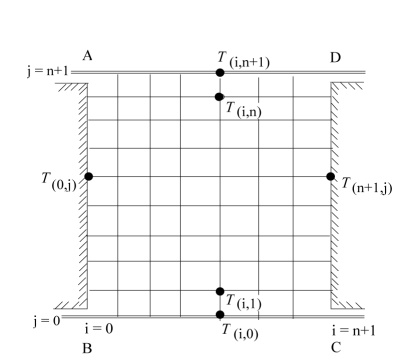Figure 3. Finite-difference grid for temperature boundary conditions

On the left plate AB:

$\xi_{0, j}=\frac{2\left(\psi_{0, j}-\psi_{1, j}+\left.\frac{\partial \psi}{\partial x}\right|_{0, j} \Delta x\right)}{\Delta x^{2}}, T_{0, j}=-0.5$

On the right plate DC:

$\xi_{n+4, j}=\frac{2\left(\psi_{n+1, j}-\psi_{n, j}-\left.\frac{\partial \psi}{\partial x}\right|_{n+1, j} \Delta x\right)}{\Delta x^{2}}, T_{n+1, j}=0.5$

$\xi_{i, n+1}=\frac{2\left(\psi_{i, n+1}-\psi_{i, n}-\left.\frac{\partial \psi}{\partial y}\right|_{k+1} \Delta y\right)}{\Delta y^{2}}, T_{i, n+1}=\frac{\Delta y+T_{i, n}}{1+\Delta y}$

On the bottom plate BC:

$\xi_{i, 0}=\frac{2\left(\psi_{i, 0}-\psi_{i, 1}+\left.\frac{\partial \psi}{\partial y}\right|_{i, 0} \Delta y\right)}{\Delta y^{2}}, T_{i, 0}=\frac{-\Delta y+T_{i, 1}}{1+\Delta y}$

The homogeneous Neumann boundary conditions for pressure are given by

$\frac{\partial P}{\partial \mathfrak{n}}=0$ ，

where n is the normal-direction. Elliptic equations with Neumann boundary conditions on all boundaries, such as the pressure Eq. (12), present an indeterminate problem, as the coefficient matrix of the finite-difference representation of the equation has one zero eigenvalue . Consequently, the resulting system of equations is linearly dependent and cannot be solved uniquely. This can be alleviated by assigning a constant value to pressure at one reference point in the solution domain. We have assigned P=5 at one reference point in the solution domain. The resulting equations will be linearly independent and will have a unique solution. Thus, the pressure solution will be off by this constant, but the pressure gradient, which is the actual term that exists in the equation of motion, will be correctly calculated.

4. Stability and Convergence of the Numerical Method

In this section, we will prove the stability and convergence of the numerical (ADI) method used in the numerical discretization based on the criteria proposed by Peaceman and Rachford . We now introduce the finite-difference formulae for first and second-order partial derivatives of ξ with respect to x and y as proposed in , which are given by

$D_{+, x}(\Delta x) \xi_{i, j}=\frac{\xi_{i+1, j}-\xi_{i, j}}{\Delta x}+O(\Delta x)$

$D_{-, x}(\Delta x) \xi_{i, j}=\frac{\xi_{i, j}-\xi_{i-1, j}}{\Delta x}+O(\Delta x)$

$D_{0, x}(\Delta x) \xi_{i, j}=\frac{\xi_{i+1, j}-\xi_{i-1, j}}{2 \Delta x}+O\left(\Delta x^{2}\right)$

Similarly, for second-order partial derivatives we will employ the second central difference,

$D_{0, x}^{2}(\Delta r) \xi_{i, j}=\frac{\xi_{i+1, j}-2 \xi_{i, j}+\xi_{i-1, j}}{\Delta x^{2}}+O\left(\Delta x^{2}\right)$

where $\Delta x$ is the grid spacing in x-direction. We adopt similar formulas in y direction. Let $\Delta t$ denotes the grid spacing in time (t)-direction. Further, we assume $A=D_{0, x}^{2}$ , $B=D_{0, y}^{2}$ , $C=D_{0, x}$ and $\mathrm{D}=D_{0 . y}$ . Now, discretizing the equation Eq. (15) at time step t+1/2 we get

$\xi_{i, j}^{t+\frac{1}{2}}-\xi_{i, j}^{t}=\frac{\Delta t}{2 R e} A \xi_{i, j}^{\xi t}-\frac{\Delta t}{2} u_{i, j} C \xi_{i, j}^{t}+\frac{\Delta t}{2 R e} B \xi_{i, j}^{t+\frac{1}{2}}-\frac{\Delta t}{2} v_{i, j} D \xi_{i, j}^{t+\frac{1}{2}}$

which gives

$\left[I+\frac{\Delta t}{2} v_{i, j}, D-\frac{\Delta t}{2 R e} B\right] \xi_{i, j}^{t+\frac{1}{2}}=\left[I-\frac{\Delta t}{2} u_{i, j} C+\frac{\Delta t}{2 R e} A\right] \xi_{i, j}^{t}$     (21)

Now, discretizing the Eq. (15) at time step t+1 we get

$\xi_{i, j}^{t+1}-\xi_{i, j}^{t+\frac{1}{2}}=\frac{\Delta t}{2 R e} A \xi_{i, j}^{t+1}-\frac{\Delta t}{2} u_{i, j} C \xi_{i, j}^{t+1}+\frac{\Delta t}{2 R e} B \xi_{i, j}^{t+\frac{1}{2}}-\frac{\Delta t}{2} v_{i, j} D \xi_{i, j}^{t+\frac{1}{2}}$

which gives

$\left[I+\frac{\Delta t}{2} u_{i, j} C-\frac{\Delta t}{2 R e} A\right] \xi_{i, j}^{t+1}=\left[I-\frac{\Delta t}{2} v_{i, j} D+\frac{\Delta t}{2 R e} B\right] \xi_{i, j}^{t+\frac{1}{2}}$   (22)

Now, equations (21) and (22) give,

$\left[I+\frac{\Delta t}{2} u_{i, j} C-\frac{\Delta t}{2 R e} A\right] \xi_{i, j}^{t+1}=\left[I-\frac{\Delta t}{2} v_{i, j} D+\frac{\Delta t}{2 R e} B\right]\left[I+\frac{\Delta t}{2} v_{i, j} D-\frac{\Delta t}{2 R e} B\right]^{-1}$ $\times\left[I-\frac{\Delta t}{2} u_{i, j} C+\frac{\Delta t}{2 R e} A\right] \xi_{i, j}^{t}$        (23)

Rewriting the equations (21), (22) and (23) we obtain the following form:

$\xi_{i, j}^{t+\frac{1}{2}}=E \xi_{i, j}^{t}$   (24)

$\xi_{i, j}^{t+1}=F \xi_{i, j}^{t+\frac{1}{2}}$   (25)

$\xi_{i, j}^{t+1}=F E \xi_{i, j}^{t}$   (26)

where

$E=\left[I+\frac{\Delta t}{2} v_{i, j} D-\frac{\Delta t}{2 R e} B\right]^{-1}\left[I-\frac{\Delta t}{2} u_{i, j} C+\frac{\Delta t}{2 R e} A\right]$       (27)

$F=\left[I+\frac{\Delta t}{2} u_{i, j} C-\frac{\Delta t}{2 R e} A\right]^{-1}\left[I-\frac{\Delta t}{2} v_{i, j} D+\frac{\Delta t}{2 R e} B\right]$       (28)

Now, discretizing the equation Eq. (16) at time step t+1/2, we get

$T_{i, j}^{t+\frac{1}{2}}-T_{i, j}^{t}=\frac{\Delta t}{2 P r} A T_{i, j}^{t}-\frac{\Delta t}{2} u_{i, j} C T_{i, j}^{t}+\frac{\Delta t}{2 P r} B T_{i, j}^{t+\frac{1}{2}}-\frac{\Delta t}{2} v_{i, j} D T_{i, j}^{t+\frac{1}{2}}$

which gives

$\left[I+\frac{\Delta t}{2} v_{i, j} D-\frac{\Delta t}{2 P r} B\right] T_{i, j}^{t+\frac{1}{2}}=\left[I-\frac{\Delta t}{2} u_{i, j} C+\frac{\Delta t}{2 P r} A\right] T_{i, j}^{t}$    (29)

Now, discretizing the equation Eq. (16) at time step t+1 we get

$T_{i, j}^{t+1}-T_{i, j}^{t+\frac{1}{2}}=\frac{\Delta t}{2 P r} A T_{i, j}^{t+1}-\frac{\Delta t}{2} u_{i, j} C T_{i, j}^{t+1}+\frac{\Delta t}{2 P r} B T_{i, j}^{t+\frac{1}{2}}-\frac{\Delta t}{2} v_{i, j} D T_{i, j}^{t+\frac{1}{2}}$

which gives

$\left[I+\frac{\Delta t}{2} u_{i, j} C-\frac{\Delta t}{2 P r} A\right] T_{i, j}^{t+1}=\left[I-\frac{\Delta t}{2} v_{i, j} D+\frac{\Delta t}{2 P r} B\right] T_{i, j}^{t+\frac{1}{2}}$    (30)

Now, equations (29) and (30) together give,

$\left[I+\frac{\Delta t}{2} u_{i, j} C-\frac{\Delta t}{2 P r} A\right]_{i, j}^{t-1}=\left[I-\frac{\Delta t}{2} v_{i, j} D+\frac{\Delta t}{2 P r} B\right]\left[I+\frac{\Delta t}{2} v_{i, j} D-\frac{\Delta t}{2 P r} B\right]^{-1}$ $\times\left[I-\frac{\Delta t}{2} u_{i, j} C+\frac{\Delta t}{2 P r} A\right] T_{i, j}^{t}$        (31)

Rewriting the equations (29), (30) and (31) we obtain the following form:

$T_{i, j}^{t+\frac{1}{2}}=E^{\prime} T_{i, j}^{t}$      (32)

$T_{i, j}^{t+1}=F^{\prime} T_{i, j}^{t+\frac{1}{2}}$          (33)

$T_{i, j}^{t+1}=F^{\prime} E^{\prime} T_{i, j}^{t}$         (34)

where

$E^{\prime}=\left[I+\frac{\Delta t}{2} v_{i, j} D-\frac{\Delta t}{2 P r} B\right]^{-1}\left[I-\frac{\Delta t}{2} u_{i, j} C+\frac{\Delta t}{2 P r} A\right]$   (35)

$F^{\prime}=\left[I+\frac{\Delta t}{2} u_{i, j} C-\frac{\Delta t}{2 P r} A\right]^{-1}\left[I-\frac{\Delta t}{2} v_{i, j} D+\frac{\Delta t}{2 P r} B\right]$   (36)

Recall, the equations (26) and (34), these equations in matrix form can be written as

$\left[\begin{array}{c}{\xi_{i, j}^{t+1}} \\ {T_{i, j}^{t+1}}\end{array}\right]=\left[\begin{array}{cc}{F E} & {0} \\ {0} & {F^{\prime} E^{\prime}}\end{array}\right]\left[\begin{array}{c}{\xi_{i, j}^{t}} \\ {T_{i, j}^{t}}\end{array}\right]$   (37)

From the matrix method for stability, we must have the norm of the above matrix must be less than or equal to 1, i.e.

$\| \begin{array}{cc}{F E} & {0} \\ {0} & {F E}\end{array} ] \| \leq 1$

which gives $\|F E\| \leq 1$ and $\left\|F^{\prime} E^{\prime}\right\| \leq 1$ . Now, recall that for any matrix M, $\rho(\mathrm{M}) \leq\|M\|$ for all matrix norms $\|s\|$ . If we now take $\|s\|$ to be the spectral norm for the matrices (and 2-norm for vectors) so $\|\cdot\|=\rho(\cdot)$ , which is valid provided E and F are diagonalizable with real eigenvalues. So, $\|F E\|=\rho(F) \rho(E)$ and $\left\|F^{\prime} E^{\prime}\right\|=\rho\left(F^{\prime}\right) \rho\left(E^{\prime}\right)$ . Thus our task reduces to show that $\rho(E) \leq 1$ and $\rho(F) \leq 1$ in order to achieve $\|F E\| \leq 1$ . Similarly, to obtain $\left\|F^{\prime} E^{\prime}\right\| \leq 1$ we need $\rho\left(F^{\prime}\right) \leq 1$ and $\rho\left(E^{\prime}\right) \leq 1$ .

Now, we will prove that $\rho(F) \leq 1$ and leave the case $\rho(F) \leq 1$ because the same result is obtained as that of $\rho(E) \leq 1$.

Consider equations (27), (28), (35) and (36) with A=B and C=D. We note that this occurs for the vorticity transport equation and the energy equation if the same spatial step size is used in both directions.

Now, writing the discretized equation of Eq. (24), we get

$\left[\frac{\Delta t}{4 \Delta y} v_{i, j}-\frac{\Delta t}{2 \operatorname{Re} \Delta y^{2}}\right] \xi_{i, j+1}^{t+\frac{1}{2}}+\left(1+\frac{\Delta t}{R e \Delta y^{2}}\right) \xi_{i, j}^{t+\frac{1}{2}}+\left[-\frac{\Delta t}{4 \Delta y} v_{i, j}-\frac{\Delta t}{2 \operatorname{Re} \Delta y^{2}}\right] \xi_{i, j-1}^{t+\frac{1}{2}}$ $=\left[-\frac{\Delta t}{4 \Delta x} u_{i, j}+\frac{\Delta t}{2 \operatorname{Re} \Delta x^{2}}\right] \xi_{i, j+1}^{t}+\left(1-\frac{\Delta t}{\operatorname{Re} \Delta x^{2}}\right) \xi_{i, j}^{t}+\left[\frac{\Delta t}{4 \Delta x} u_{i, j}+\frac{\Delta t}{2 \operatorname{Re} \Delta x^{2}}\right] \xi_{i, j-1}^{t}$   (38)

If the boundary values at i = 0, i = n+1, j = 0 and j = n+1 are known, then for a fixed i in x-direction and varying the values for j in y direction, j = 1(1)n, we obtain a system of equations. We can write the above system of equations in matrix form as follows:

$\left[\begin{array}{ccccc}{a} & {b} & {} & {} & {} \\ {c} & {a} & {b} & {} & {} \\ {} & {\cdot} & {\cdot} & {\cdot} \\ {} & {} & {c} & {a} & {b} \\ {} & {} & {} & {c} & {a}\end{array}\right]$ $\left[\begin{array}{c}{\xi_{i, j_{1}}^{t+\frac{1}{2}}} \\ {\xi_{i, j_{2}}^{t+\frac{1}{2}}} \\ {\vdots} \\ {\xi_{i, j_{n}}^{t+\frac{1}{2}}}\end{array}\right]$ = $\left[\begin{array}{ccccc}{a^{\prime}} & {b^{\prime}} & {} & {} & {} \\ {c^{\prime}} & {a^{\prime}} & {b^{\prime}} & {} & {} \\ {} & {\cdot} & {\cdot} & {\cdot} & {} \\ {} & {} & {c^{\prime}} & {a^{\prime}} & {b^{\prime}} \\ {} & {} & {} & {c^{\prime}} & {a^{\prime}}\end{array}\right]$ $\left[\begin{array}{c}{\xi_{i, j_{1}}^{t}} \\ {\xi_{i, j_{2}}^{t_{i}}} \\ {\vdots} \\ {\xi_{i, j_{n}}^{t_{i}}}\end{array}\right]$ $+\boldsymbol{g}_{j}$

where

$a=\left[1+\frac{\Delta t}{R e \Delta y^{2}}\right], a^{\prime}=\left[1-\frac{\Delta t}{R e \Delta x^{2}}\right]$

$b=\left[\frac{\Delta t}{4 \Delta y} v_{i, j}-\frac{\Delta t}{2 \operatorname{Re} \Delta y^{2}}\right], b^{\prime}=\left[-\frac{\Delta t}{4 \Delta x} u_{i, j}+\frac{\Delta t}{2 \operatorname{Re} \Delta x^{2}}\right]$

$c=\left[-\frac{\Delta t}{4 \Delta y} v_{i, j}-\frac{\Delta t}{2 \operatorname{Re} \Delta y^{2}}\right], c^{\prime}=\left[\frac{\Delta t}{4 \Delta x} u_{i, j}+\frac{\Delta t}{2 \operatorname{Re} \Delta x^{2}}\right]$

and gj is a column vector of known boundary values of $\xi_{i, j_{0}}^{t+\frac{1}{2}}$ , $\xi_{i, j_{n+1}}^{t+\frac{1}{2}}$ at time t+1/2, and zeros. Rewriting the above system as follows

$\mathcal{P}_{1} \zeta^{t+\frac{1}{2}}=Q_{1} \zeta^{t}+\mathcal{R}_{1}^{t+\frac{1}{2}}$

where the matrices $\mathcal{P}_{1}$ , $\mathcal{Q}_{1}$  are of order n as shown above, $\zeta^{t+\frac{1}{2}}$ and $\zeta^{t}$ denote the column vectors with components

$\xi_{i, j_{1}}^{t+\frac{1}{2}}, \xi_{i, j_{2}}^{t+\frac{1}{2}}, \ldots \xi_{i, j_{n}}^{t+\frac{1}{2}}, \quad$ and $\quad \xi_{i, j_{1}}^{\xi t}, \xi_{i, j_{2}}^{\xi t}, \ldots \xi_{i, j_{n}}^{t}$ respectively, $\mathcal{R}_{1}^{t+\frac{1}{2}}$ denotes the column vector of known boundary values of  $\xi_{i, j_{0}}^{t+\frac{1}{2}}$ , $\xi_{i, j_{n+1}}^{t+\frac{1}{2}}$ at time t+1/2, and zeros. Now, calculating the eigenvalues of matrix $\mathcal{P}_{1}$ ,

$b c=\left[\frac{\Delta t}{4 \Delta y} v_{i, j}-\frac{\Delta t}{2 R e \Delta y^{2}}\right]\left[-\frac{\Delta t}{4 \Delta y} v_{i, j}-\frac{\Delta t}{2 R e \Delta y^{2}}\right]$ $=\frac{\Delta t^{2}}{4 R e^{2} \Delta y^{4}}\left[1-\frac{v_{i, j}^{2} \Delta y^{2} R e^{2}}{4}\right]$

So, the eigenvalues of matrix $\mathcal{P}_{1}$ are

$\lambda_{p s}=\left(1+\frac{\Delta t}{\operatorname{Re} \Delta y^{2}}\right)+\frac{\Delta t}{\operatorname{Re} \Delta y^{2}}\left(\sqrt{1-\frac{v_{i, j}^{2} \Delta y^{2} R e^{2}}{4}}\right) \cos \left(\frac{s \pi}{n+1}\right)$

where $s$= 1$(1) n$. Now, calculating the eigenvalues of matrixQ1,

$b^{\prime} c^{\prime}=\left[-\frac{\Delta t}{4 \Delta x} u_{i, j}+\frac{\Delta t}{2 \operatorname{Re} \Delta x^{2}}\right]\left[\frac{\Delta t}{4 \Delta x} u_{l, j}+\frac{\Delta t}{2 \operatorname{Re} \Delta x^{2}}\right]$ $=\frac{\Delta t^{2}}{4 R e^{2} \Delta x^{4}}\left[1-\frac{u_{i, j}^{2} \Delta x^{2} R e^{2}}{4}\right]$

Therefore, the eigenvalues of matrix Q1 are

$\lambda_{q s}=\left(1-\frac{\Delta t}{\operatorname{Re} \Delta x^{2}}\right)+\frac{\Delta t}{\operatorname{Re} \Delta x^{2}}\left(\sqrt{1-\frac{u_{i, j}^{2} \Delta x^{2} R e^{2}}{4}}\right) \cos \left(\frac{s \pi}{n+1}\right)$

where s=1(1)n. Thus, for the stability we must have

$\left\|\mathcal{P}_{1}^{-1} Q_{1}\right\|_{2}=\rho\left(\mathcal{P}_{1}^{-1} \mathcal{Q}_{1}\right)=\max _{s}\left|\frac{\lambda_{q s}}{\lambda_{p s}}\right| \leq 1, \quad s=1(1) n$

$\left\|\mathcal{P}_{1}^{-1} \mathcal{Q}_{1}\right\|_{2}=\max _{s}\left|\frac{\left(1-\frac{\Delta t}{R e \Delta x^{2}}\right)+\frac{\Delta t}{R e \Delta x^{2}}\left(\sqrt{1-\frac{u_{i, j}^{2} \Delta x^{2} R e^{2}}{4}}\right) \cos \left(\frac{s \pi}{n+1}\right)}{\left(1+\frac{\Delta t}{R e \Delta y^{2}}\right)+\frac{\Delta t}{R e \Delta y^{2}}\left(\sqrt{1-\frac{v_{i, j}^{2} \Delta y^{2} R e^{2}}{4}}\right) \cos \left(\frac{s \pi}{n+1}\right)}\right| \leq 1$        (39)

We have chosen

$\Delta x=\Delta y, r=\frac{\Delta t}{\operatorname{Re} \Delta x^{2}}=\frac{\Delta t}{\operatorname{Re} \Delta y^{2}}, M=\frac{u_{i, j}^{2} \Delta x^{2} R e^{2}}{4}$ and $M^{\prime}=\frac{v_{i, i}^{2} \Delta y^{2} R e^{2}}{4}$

Then the Eq. (39) can be expressed in the form

$\left\|\mathcal{P}_{1}^{-1} \mathcal{Q}_{1}\right\|_{2}=\max _{s}\left|\frac{1-r+r \sqrt{1-M} \cos \left(\frac{s \pi}{n+1}\right)}{1+r+r \sqrt{1-M^{\prime}} \cos \left(\frac{s \pi}{n+1}\right)}\right| \leq 1, \quad s=1(1) n$       (40)

It is easy to observe that $|M| \leq 1,\left|M^{\prime}\right| \leq 1$ or

$\left|\frac{u_{i, j}^{2} \Delta x^{2} R e^{2}}{4}\right| \leq 1,\left|\frac{v_{i, j}^{2} \Delta y^{2} R e^{2}}{4}\right| \leq 1$.

Thus, we have $\left|u_{i, j} \Delta x R e\right| \leq 2$ and $\left|v_{i, j} \Delta y R e\right| \leq 2$ . Also letting

$f=\frac{1-r+r \sqrt{1-M} \cos \left(\frac{s \pi}{n+1}\right)}{1+r+r \sqrt{1-M^{\prime}} \cos \left(\frac{s \pi}{n+1}\right)}, \quad$ we see that $\quad \frac{d f}{d s}=0$ gives $s=n+1$

For this value of s, $\left\|\mathcal{P}_{1}^{-1} \mathcal{Q}_{1}\right\|_{2}$ has maximum value, therefore Eq. (40) reduces to

$\left|\frac{1-r-r \sqrt{1-M}}{1+r-r \sqrt{1-M^{\prime}}}\right| \leq 1$

which gives

$r \leq \frac{2}{\left[\sqrt{1-M^{\prime}}+\sqrt{1-M}\right]}<1$    (41)

Since $|M| \leq 1,\left|M^{\prime}\right| \leq 1$ , also

$r=\frac{\Delta t}{R e \Delta x^{2}}=\frac{\Delta t}{R e \Delta y^{2}} \geq 0$   (42)

Thus, from equations (41) and (42) we have $0 \leq r<1$ i.e. $\Delta t<R e \Delta x^{2}$ Similarly, we will prove that $\rho(E) \leq 1$ and leave the case $\rho\left(F^{\prime}\right) \leq 1$ because the same result is obtained as that of $\rho(E) \leq 1$. Now, $E^{\prime}$ is equivalent to the following matrix system:

$\left[\begin{array}{cccc}{d} & {e} & {} & {} \\ {f} & {d} & {e} & {} \\ {} & {\cdot} & {\cdot} & {\cdot} \\ {} & {} & {f} & {d} & {e} \\ {} & {} & {} & {f} & {d}\end{array}\right]$ $\left[\begin{array}{c}{T_{i, j_{1}}^{t+\frac{1}{2}}} \\ {T_{i, j_{2}}^{t+\frac{1}{2}}} \\ {\vdots} \\ {T_{i, j_{n}}^{t+\frac{1}{2}}}\end{array}\right]$  $=\left[\begin{array}{cccc}{d^{\prime}} & {e^{\prime}} & {} & {} \\ {f^{\prime}} & {d^{\prime}} & {e^{\prime}} & {} & {} \\ {} & {\cdot} & {\cdot} & {\cdot} & {} \\ {} & {} & {f^{\prime}} & {d^{\prime}} & {e^{\prime}} \\ {} & {} & {} & {f^{\prime}} & {d^{\prime}}\end{array}\right]\left[\begin{array}{c}{T_{t, j_{2}}^{t}} \\ {T_{t, j_{2}}^{t}} \\ {\vdots} \\ {T_{i, j_{n}}^{t}}\end{array}\right]+g_{j}^{\prime}$

where

$d=\left[1+\frac{\Delta t}{\operatorname{Pr} \Delta y^{2}}\right], d^{\prime}=\left[1-\frac{\Delta t}{\operatorname{Pr} \Delta x^{2}}\right]$

$e=\left[\frac{\Delta t}{4 \Delta y} v_{i, j}-\frac{\Delta t}{2 P r \Delta y^{2}}\right], e^{\prime}=\left[-\frac{\Delta t}{4 \Delta x} u_{i, j}+\frac{\Delta t}{2 P r \Delta x^{2}}\right]$

$f=\left[-\frac{\Delta t}{4 \Delta y} v_{i, j}-\frac{\Delta t}{2 P r \Delta y^{2}}\right], f^{\prime}=\left[\frac{\Delta t}{4 \Delta x} u_{i, j}+\frac{\Delta t}{2 P r \Delta x^{2}}\right]$

and $g_{j}^{\prime}$ is a column vector of known boundary values of $T_{i, j_{0}}^{t+\frac{1}{2}}$ , $T_{i, j_{n+1}}^{t+\frac{1}{2}}$ at time t+1/2, and zeros. Rewriting  the above system as follows

$\mathcal{P}_{2} \theta^{t+\frac{1}{2}}=\mathcal{Q}_{2} \theta^{t}+\mathcal{R}_{2}^{t+\frac{1}{2}}$

where the matrices $\mathcal{P}_{2}$ , $\mathcal{Q}_{2}$ are of order $n$ as shown above, $\theta^{t+\frac{1}{2}}$ and $\theta^{t}$ denote the column vectors with components $T_{i, j_{1}}^{t+\frac{1}{2}}, T_{i, j_{2}}^{t+\frac{1}{2}}, \ldots T_{i, j_{n}^{2}}^{t+\frac{1}{2}}$ and $\quad T_{i, j_{1}}^{t}, T_{i, j_{2}}^{t}, \ldots T_{i, j_{n}}^{t}$ respectively, $R_{2}^{t+\frac{1}{2}}$ denotes the column vector of known boundary values of $T_{i, j_{0}}^{t+\frac{1}{2}}$ , $T_{i, j_{n+1}}^{t+\frac{1}{2}}$ at time t+1/2, and zeros. Now, preceding as for the above case, we get $\Delta t<P r \Delta x^{2}$ Thus, we have proved that

$\left|u_{i, j} \Delta x R e\right| \leq 2,\left|v_{i, j} \Delta y R e\right| \leq 2, \quad \Delta t<R e \Delta x^{2}$

$\left|u_{i, j} \Delta x P r\right| \leq 2,\left|v_{i, j} \Delta y P r\right| \leq 2, \quad \Delta t<P r \Delta x^{2}$

Hence, the numerical scheme we have used is unconditionally stable. The scheme is consistent as the limiting value of the local truncation is zero as $\Delta t$ , $\Delta x$ and $\Delta y \rightarrow 0$ . So, by Lax's equivalence theorem [26, pp.72], the given scheme is convergent.

5. Numerical Computations

We obtained the numerical computation of the unknown flow variables $\psi$ , $\xi$ , $u$ , $v$ , $P$ , $T$ for the present problem with the aid of a computer programme developed and run in C++ compiler. The input data for the relevant parameters in the governing equations like Reynolds number (Re) and Prandtl number (Pr) have been properly chosen incompatible with the present problem considered.

5.1 Algorithm for obtaining numerical solutions by streamfunction-vorticity formulation

The algorithm for obtaining the numerical solutions by streamfunction-vorticity formulation consists of the following steps:

Step (1) Specify the initial values for $\xi$ and $\psi$ at time $t=0$.

Step (2)Solve the vorticity transport equation Eq. (7) for $\xi$ at each interior grid point at time $t+\Delta t$ .

Step (3)  Iterate for new $\psi$ values at all points by solving the Poisson equation Eq. (9) using newξ values at interior points.

Step (4) Find the velocity components  and $v=-\frac{\partial \psi}{\partial x} \cdot u=\frac{\partial \psi}{\partial y}$

Step (5) Solve the energy equation Eq. (10) for T at each interior grid point using $u$ and $v$ values.

Step (6) Find the local Nusselt-number $N u_{y}$ = $\frac{\partial T}{\partial x}$ and average Nusselt-number $\overline{N u}$ .

Step (7) Solve the Poisson equation Eq. (12) for P using the calculated $\psi$ values at each interior grid point.

Step (8) Determine the values of ξ on the boundaries using $\psi$ and ξ values at interior points.

Step (9) Return to Step 2 if the solution does not converge.

6. Numerical Results and Discussion

We used the Alternating-Direction-Implicit (ADI) scheme to carry out the numerical computations of the unknown flow variables $\psi$ , $\xi$ , $u$ , $v$, $P$ , $T$ for the present problem. We summarized this method under an algorithm for streamfunction-vorticity formulation as described under section 5.1 above. We used a computer code developed and run in C++ compiler to execute this. To verify our computer code, the numerical results obtained by the present method were compared with the benchmark results reported in . It is seen that the results obtained in the present work are in good agreement with those reported in  at low Reynolds number Re=100. This indicates the validity of the numerical code that we developed.

Figures 4 and 5 illustrate the variation of $u$ -velocity along the vertical line through geometric center of the square cavity at different time levels (t=0.25sec, 0.50 sec, 0.75 sec, 1.00 sec), for different Reynolds numbers Re=15 and 50 respectively. We observed that, the u-velocity is almost same near the top and bottom wall of the square cavity, above and below the geometric center respectively for Re=15 and 50. We also observed that, the absolute value of u-velocity first decrease, then increases, and finally, decreases in the vicinity of the top wall and the same behavior is observed below the geometric center.

Figures 6 and 7 illustrate the variation of v-velocity along the horizontal line through geometric center of the square cavity at different time levels (t=0.25sec, 0.50 sec, 0.75 sec, 1.00 sec), for different Reynolds numbers Re=15 and 50 respectively. We observed that, the absolute value of v-velocity first increases uniformly, and finally, converge to the boundary of the right wall. The behavior of v-velocity from the left side wall towards the geometric center of the cavity is that it increases uniformly, and converges at the geometric center of the cavity. We also observed that, the absolute value of v-velocity increases uniformly as time increases, and converges at the geometric center, and the same behavior is also observed to the right side of the geometric center at both Reynolds numbers Re=15 and 50.

## 4.png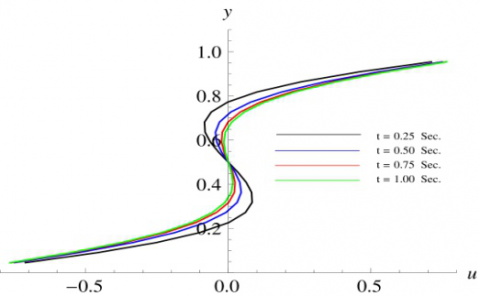Figure 4. u-velocity profiles along the vertical line through geometric center of the square cavity for Re=15 at different time levels

## 5.png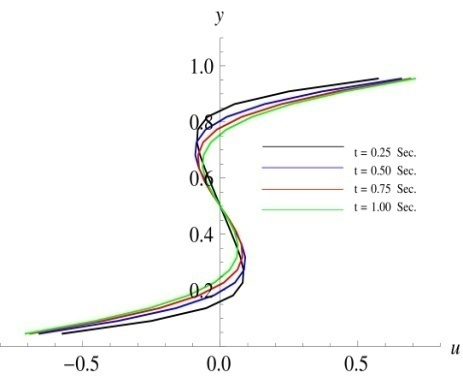Figure 5. u-velocity profiles along the vertical line through geometric center of the square cavity for Re=50 at different time levels

## 6.png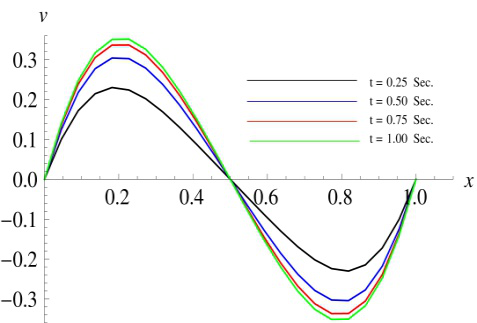Figure 6. v-velocity profiles along the horizontal line through geometric center of the square cavity for Re=15 at different time levels

Figures 8 and 9 illustrate the variation of pressure along the horizontal line through geometric center of the square cavity at different time levels (t = 0.25 sec, 0.50 sec, 0.75 sec, 1.00 sec), for different Reynolds numbers Re=15 and 50 respectively. We observed that, the pressure first increases, then decreases in between the left wall boundary to the mid of the square domain and the same behavior is observed to the right of geometric center for Re=15 at $t$ =1 sec. We found that the pressure first increases, then decreases, and finally, increases in between left wall boundary and geometric center of the square cavity while it decreases, increases, then decreases, and finally, increases to the right of the geometric center of the square domain for Re=50 at $t$ =1 sec.

## 7.png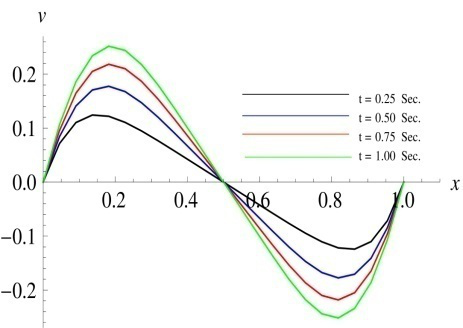Figure 7. v-velocity profiles along the horizontal line through geometric center of the square cavity for Re=50 at different time levels

## 8.png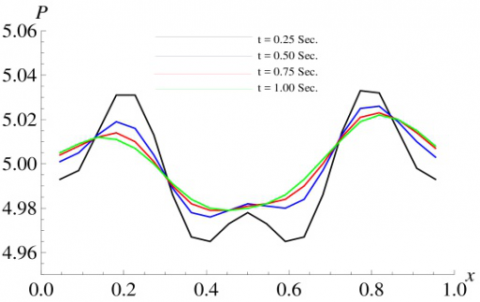Figure 8. Pressure along the horizontal line through geometric center of the square cavity for Re=15 at different time levels

## 9.png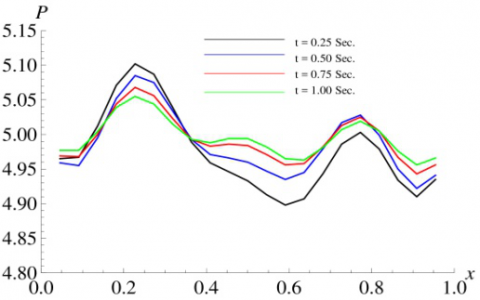Figure 9. Pressure along the horizontal line through geometric center of the square cavity for Re=50 at different time levels

Figures 10 and 11 illustrate the variation of pressure along the vertical line through geometric center of the square cavity at different time levels (t = 0.25 sec, 0.50 sec, 0.75 sec, 1.00 sec), for different Reynolds numbers Re=15 and 50 respectively. We observed that, the pressure first decreases, then increases, and finally, decreases in between bottom wall boundary and the geometric center of the square cavity, while it first increases, then decreases, increases, and finally, decreases in between the top wall and the geometric center of the square cavity for Reynolds numbers Re=15. We found that the pressure-profiles for Re=50, in between the bottom wall boundary and the geometric center of the square cavity are same as that of Re=15, while it first decreases, then increases and finally decreases in between the top wall boundary and mid of the square domain.

## 10.png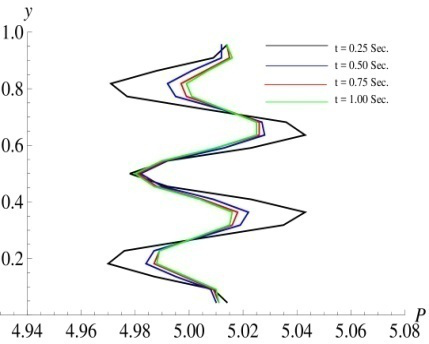Figure 10. Pressure along the vertical line through geometric center of the square cavity for Re=15 at different time levels

## 11.png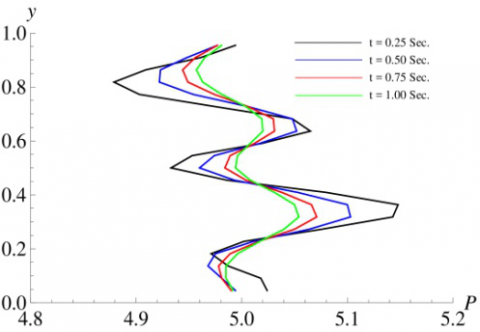Figure 11. Pressure along the vertical line through geometric center of the square cavity for Re=50 at different time levels

## 12.pngFigure 12. Temperature-profiles along the horizontal line through geometric center of the square cavity for Re=15 and Pr= 0.7 at different time levels

## 13.png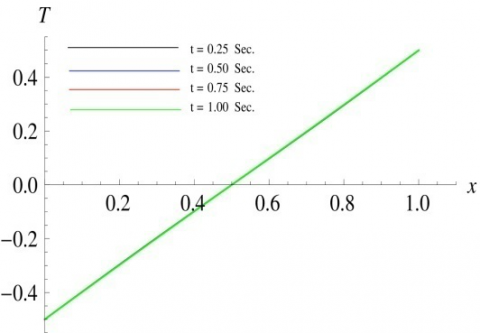Figure 13. Temperature-profiles along the horizontal line through geometric center of the square cavity for Re=50 and Pr= 0.7 at different time levels

Figures 12 and 13 illustrate the variation of temperature-profiles along the horizontal line through geometric center of the square cavity at different time levels (t = 0.25 sec, 0.50 sec, 0.75 sec, 1.00 sec), for different Reynolds numbers Re=15, 50and Prandtl number Pr=0.7 (for air) respectively. We observed that, at a particular time level, the absolute value of temperature decreases in the vicinity of right wall and the same behavior is observed to the left of geometric center of the cavity. We also observed that, the absolute value of temperature increases uniformly as time increases for both Reynolds numbers Re=15 and 50.

Figures 14 and 15 illustrate the variation of temperature-profiles along the vertical line through geometric center of the square cavity at different time levels for different Reynolds numbers Re=15, 50 and Prandtl number Pr=0.7 (for air) respectively. We observed that, the absolute value of temperature decreases in the vicinity of top wall and the same behavior is observed below the geometric center of the cavity. We also observed that, the absolute value of temperature decreases uniformly as time increases for both Reynolds number Re=15 and 50.

Figures 16 and 17 illustrate the variation of temperature-profiles along the horizontal line through geometric center of the square cavity at different time levels for different Reynolds numbers Re=15, 50 and Prandtl number Pr=6.75 (for water) respectively. We observed that, at a particular time level, the absolute value of temperature decreases in the vicinity of right wall and same behavior is observed to the left of the geometric center of the cavity. We also observed that, the absolute value of temperature increases uniformly as time increases for both Reynolds number Re=15 and 50.

## 14.png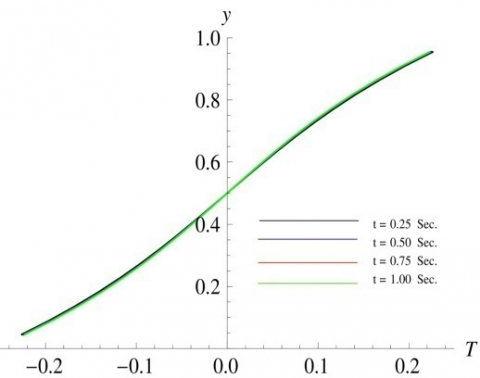Figure 14. Temperature-profiles along the vertical line through geometric center of the square cavity for Re=15 and Pr=0.7 at different time levels

## 15.png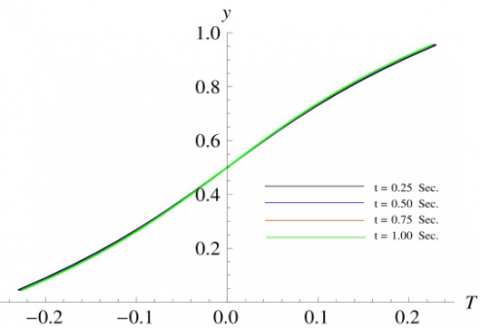Figure 15. Temperature-profiles along the vertical line through geometric center of the square cavity for Re=50 and Pr= 0.7 at different time levels

## 16.png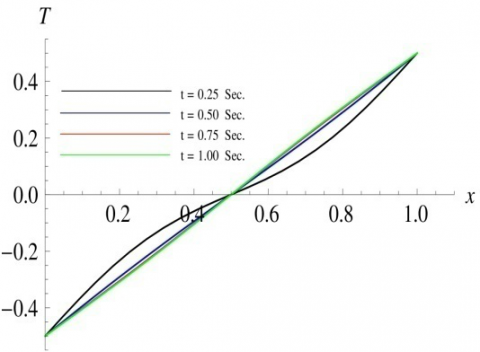Figure 16. Temperature-profiles along the horizontal line through geometric center of the square cavity for Re=15 and Pr= 6.75 at different time levels

## 17.png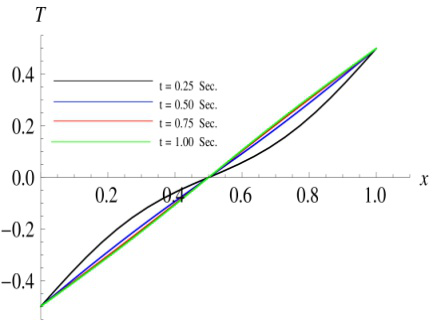Figure 17. Temperature-profiles along the horizontal line through geometric center of the square cavity for Re=50 and Pr= 6.75 at different time levels

Figures 18 and 19 illustrate the variation of temperature-profiles along the vertical line through geometric center of the square cavity at different time levels for different Reynolds numbers Re=15, 50 and Prandtl number Pr=6.75 (for water) respectively. We observed that, the absolute value of temperature decreases in the vicinity of top wall and the same behavior is observed below the geometric center of the cavity. We also observed that, the absolute value of temperature first increases uniformly, and then, decreases as time increases for both Reynolds number Re=15 and 50.

## 18.png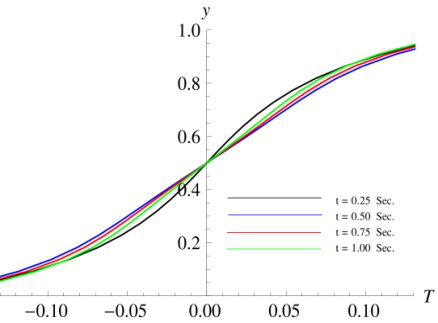Figure 18. Temperature-profiles along the vertical line through geometric center of the square cavity for Re=15 and Pr=6.75 at different time levels

## 19.png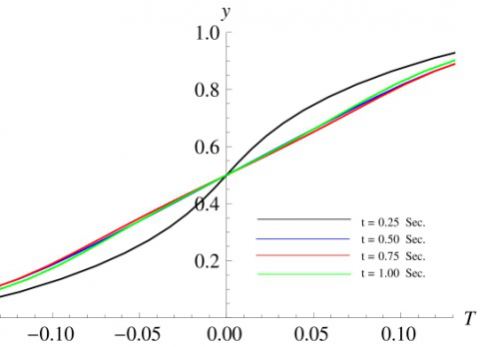Figure 19. Temperature-profiles along the vertical line through geometric center of the square cavity for Re=50 and Pr= 6.75 at different time levels

The Isotherms for Reynolds number 15, Prandtl numbers Pr=0.7 and 6.75 at different time levels ( t = 0.25 sec, 0.50 sec, 0.75 sec, 1.00 sec), have been depicted in Figures 20 and 21 respectively. Isotherms are the lines which connect points of equal temperature. The above mentioned figures show a number of curves with a particular number on it, which represent the temperature value on that curve. The isotherms labeled as -0.4 and 0.4 in both Figures 20 and 21 indicate the coldest and hottest temperature-profiles. The relative spacing of isotherms indicate the temperature gradient which is 0.1 as shown in figures, the amount by which the temperature vary across each unit of horizontal distance in a direction perpendicular to the isotherms.

## 20.png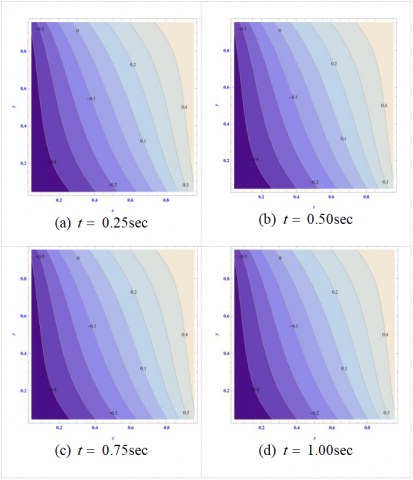Figure 20. Isotherms for Re=15 and Pr=0.7 at different time levels: (a) t = 0.25 sec; (b) t = 0.50 sec; (c) t = 0.75 sec; (d) t = 1.00 sec

## 21.png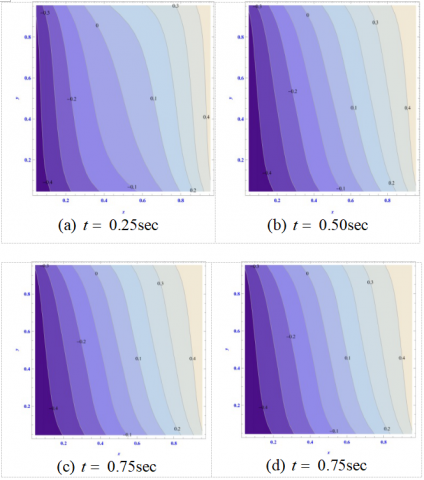Figure 21. Isotherms for Re=15 and Pr=6.75 at different time levels: (a) t = 0.25 sec; (b) t = 0.50 sec; (c) t = 0.75 sec; (d) t = 1.00 sec

The Isobars for Reynolds numbers 15 and 50 at different time levels ( $t=$ 0.25sec, 0.50 sec, 0.75 sec, 1.00sec), have been depicted in Figures 22 and 23 respectively. Isobars are the lines which connects points of equal pressure. Figures 22 and 23 shows a number of curves with a particular number on it, which represent the pressure value on that curve. We found that the maximum value of pressure is 5.1 and 5.2 for Re=15 and 50 respectively. In Figures 22 and 23, a small blue color contour shows the smallest value of pressure.

The physical quantity of interest, the Nusselt number (Nu), represents heat transfer. The local Nusselt number  $\left(N u_{y}\right)$  and average Nusselt number $\overline{N u}$ at the center line (x=0.5) of the square cavity is defined as $N u_{y}=\frac{\partial T}{\partial X}$ and $\overline{N u}=\int_{0}^{1} N u_{y} d y$ . Figure 24 illustrates the numerical computations of average Nusselt number variation, at different time-levels, along vertical line through geometric center of the square cavity. This variation shows that heat transfer rising to a peak value and subsequently converging to the steady state value. Initially transient flow dominates. After some passage of time $(t=1.0 \mathrm{sec})$ , it is evident that transient flow settles down to steady state flow.

## 22.png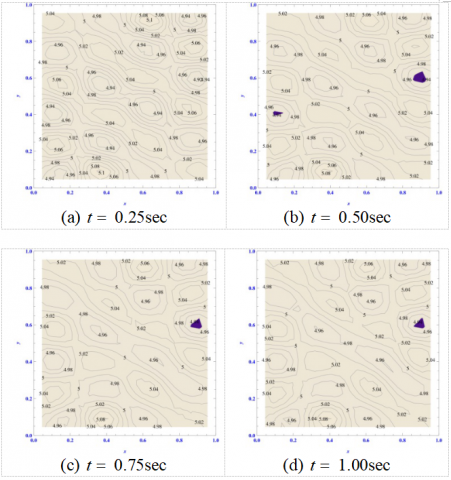Figure 22. Isobars for Re=15 at different time levels: (a) t = 0.25 sec; (b) t = 0.50 sec; (c) t = 0.75 sec; (d) t = 1.00 sec

## 23.pngFigure 23. Isobars for Re=50 at different time levels: (a) t = 0.25 sec; (b) t = 0.50 sec; (c) t = 0.75 sec; (d) t = 1.00 sec

## 24.png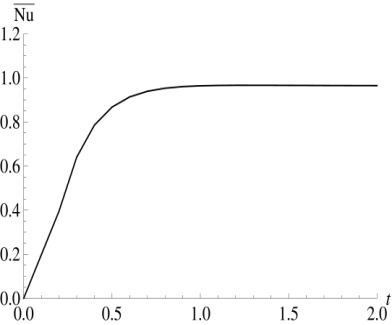Figure 24.Temporal variation of the average Nusselt number at center line $x$ = 0.5 of square cavity for Re=15 and Pr=6.75

7. Code Validation

To check the validity of our present computer code used to obtain the numerical results of u-velocity and v-velocity, we have compared our present results with those benchmark results given by Ghia et al.  and it has been found that they are in good agreement

## 25.png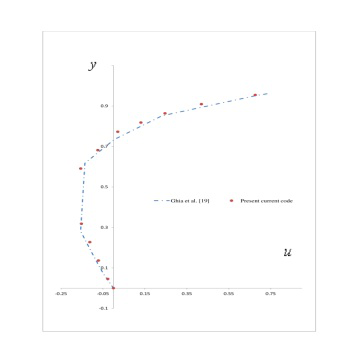Figure 25. Comparison of the numerical results of u-velocity along the vertical line through geometric center of the square cavity for Re=100

## 26.png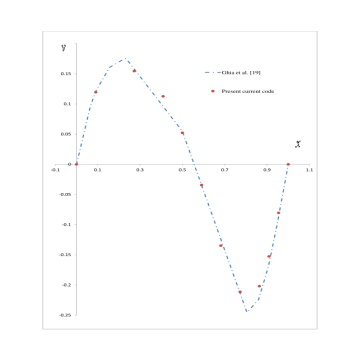Figure 26. Comparison of the numerical results of v-velocity along the horizontal line through geometric center of the square cavity for Re=100

8. Conclusions

Based on the numerical computations of velocity components pressure and temperature, we found that with the increase of Reynolds number, the absolute value of velocity components decreases and the absolute value of pressure also decreases. Further, the numerical solutions of the temperature show that, with the increase of Prandtl number, the temperature decreases along the vertical line through the geometric center of the square cavity for a particular value of Reynolds number while the temperature increases along the horizontal line through the geometric center of the square cavity for a particular value of Reynolds number.

The average Nusselt number variation along vertical line through geometric center of the square cavity for Re=15 and Pr=6.75 shows that heat transfer rising to a peak value and subsequently converging to the steady state value. Initially transient flow dominates. After some passage of time $(t=1.0 \mathrm{sec})$ , it is evident that transient flow settles down to steady state flow.

The isotherms show that the coldest and hottest temperature-profiles are -0.4 and 0.4. The relative spacing of isotherms indicate the temperature gradient, which is 0.1 as shown in figures, the amount by which the temperature vary across each unit of horizontal distance in a direction perpendicular to the isotherms. The isobars for Reynolds numbers 15 and 50 at different time levels ( $t=$ 0.25sec, 0.50sec, 0.75sec,1.00sec), are shown in the figures. We found that, the maximum value of pressure is 5.1 and 5.2 for Re=15 and 50 respectively.

Acknowledgements

The first and second authors acknowledge the financial support received from the University of Delhi under Research and Development Scheme2015-16 for providing research grant vide letter no.RC/2015/9677 dated $15^{t h}$ October 2015 as well as Non-Net Fellowship to M.phil. Scholars vide letter no.Ref.NO.Schs/Non-Net/139/EXT-145/2015-16/254dated 11 April,2015.Authors would like to convey best regards to Prof. Enrico Lorenzini for giving remarks for improving the quality of this manuscript. We Thank you so much for that.

References

 Abourida B., Hasnaoui M., Douamna S. (1999). Transient natural convection in a square enclosure with horizontal walls submitted to periodic temperatures, Numerical Heat Transfer, Part A, Vol. 36, pp. 737-750. DOI: 10.1080/104077899274543

 Alleborn N., Raszillier H., Durst F. (1999). Lid-driven cavity with heat and mass transport, Int. J. Heat Mass Transfer, Vol. 42, pp. 833-853. DOI: 10.1016/S0017-9310(98)00224-5

 Bilgen E., Oztop H. (2005). Natural convection heat transfer in partially open inclined square cavities, Int. J. Heat Mass Transfer, Vol. 48, pp. 1470-1479. DOI: 10.1590/S0104-66322007000300007

 Biringen S., Chow C.Y. (2011). An Introduction to Computational Fluid Mechanics by Examples, John Wiley and Sons, Inc., Hoboken, New Jersey.

 Chapra S.C., Canale R.P. (1989). Numerical Methods for Engineers, McGraw-Hill companies, New York.

 Chattopadhyay A., Pandit S.K., Sarma S.S., Pop I. (2016). Mixed convection in a double lid-driven sinusoidally heated porous cavity, Int. J. Heat Mass Transfer, Vol. 93, pp. 361-378. DOI: 10.1016/j.ijheatmasstransfer.2015.10.010

 Dhiman A.K., Chhabra R.P., Eswaran V. (2005). Flow and heat transfer across a confined square cylinder in the steady flow regime: Effect of Peclet number, Int. J. Heat Mass Transfer, Vol. 48, pp. 4598-4614. DOI: 10.1016/j.ijheatmasstransfer.2005.04.033

 Ghia U., Ghia K.N., Shin C.T. (1982). High-Re solutions for incompressible flow using the Navier-Stokes equations and a multigrid method, J. Comput Phys, Vol.48, pp. 387-411. DOI: 10.1.1.413.9651

 Iliev O.P., Makarov M.M. (1995). A block-matrix iterative numerical method for coupled solving 2D navier-stokes equations, J. Comput Phys, Vol. 121, pp. 324-330. DOI: 10.1016/S0021-9991(95)90170-1

 Ismael M.A., Pop I., Chamkha A.J. (2014). Mixed convection in a lid-driven square cavity with partial slip, INT J THERM, Vol. 82, pp. 47-61. DOI: 10.1.1.679.9003

 Khanafer K.M., Al-Amiri A.M., Pop I. (2007). Numerical simulation of unsteady mixed convection in a driven cavity using an externally excited sliding lid, Eur. J. Mech. B/Fluids, Vol. 26, pp. 669-687. DOI: 10.1016/j.euromechflu.2006.06.006

 Luo W.J., Yang R.J. (2007). Multiple fluid flow and heat transfer solutions in a two-sided lid-driven cavity, Int. J. Heat Mass Transfer, Vol. 50, pp. 2394-2405.

 Malleswaran A., SivasankaranS. (2016). A numerical simulation on MHD mixed convection in a lid-driven cavity with corner heaters, J. Appl. Fluid Mech., Vol. 9, No. 1, pp. 311-319.

 McDonough J.M. (2007). Lectures on computational numerical analysis of partial differential equations, from www.engr.uky.edu/~acfd/me690-lctr-nts.pdf.

 Noor D.Z., Kanna P.R., Chern M.J. (2009). Flow and heat transfer in a driven square cavity with double-sided oscillating lids in anti-phase, Int. J. Heat Mass Transfer, Vol. 52, pp. 3009-3023.

 Nouanegue H., Muftuoglu A., Bilgen E. (2008). Conjugate heat transfer by natural convection, conduction and radiation in open cavities, Int. J. Heat Mass Transfer, Vol. 51, pp. 6054-6062. DOI: 10.1016/j.ijheatmasstransfer.2008.05.009

 Oztop H.F., Dagtekin I. (2004).  Mixed convection in two-sided lid-driven differentially heated square cavity, Int. J. Heat Mass Transfer, Vol. 47, pp. 1761-1769. DOI: 10.1016/j.ijheatmasstransfer.2003.10.016

 Pandit S.K., Chattopadhyay A., Oztop H.F. (2016). A fourth order compact scheme for heat transfer problem in porous media, Computers and Mathematics with Applications, Vol. 71, pp. 805-832. DOI: 10.1016/j.camwa.2015.12.037

 Peaceman D.W., Rachford H.H. (1955). The numerical solution of parabolic and elliptic differential equations, J. Soc. Ind. Appl. Math., Vol.3, pp. 28-41. DOI: 10.1137/0103003

 Rahman M.M., Alim M.A., Mamun M.A.H., Chowdhury M.K., Islam A.K.M.S. (2007). Numerical study of opposing mixed convection in a vented enclosure, ARPN Journal of Engineering and Applied Sciences, Vol. 2, No. 2.

 Rubin S.G., Khosla P.K. (1981). Navier-stokes calculations with a coupled strongly implicit method, Computers and Fluids, Vol. 9, pp. 163-180. DOI: 10.1016/0045-7930(81)90023-2

 Sivasankaran S., Malleswaran A., Lee J., Sundar P. (2011). Hydro-magnetic combined convection in a lid-driven cavity with sinusoidal boundary conditions on both sidewalls, Int. J. Heat Mass Transfer, Vol. 54, pp. 512-525. DOI: 10.1016/j.ijheatmasstransfer.2010.09.018

 Sivasankaran S., Pan K.L. (2012). Numerical simulation on mixed convection in a porous lid-driven cavity with non uniform heating on both side walls, Numerical Heat Transfer, Part A: Applications: An International Journal of Computation and Methodology, Vol. 61, No. 2, pp. 101-121.

 Sivasankaran S., Sivakumar V., Hussein A.K. (2013). Numerical study on mixed convection in an inclined lid-driven cavity with discrete heating, Int Commun Heat Mass, Vol. 46, pp. 112-125. DOI: 10.1016/j.icheatmasstransfer.2013.05.022

 Sivasankaran S., Sivakumar V., Prakash P. (2010). Numerical study on mixed convection in a lid-driven cavity with non-uniform heating on both sidewalls, Int. J. Heat Mass Transfer, Vol. 53, pp. 4304-4315. DOI: 10.1016/j.ijheatmasstransfer.2010.05.059

 Smith G.D. (1985). Numerical Solution of Partial Differential Equations: Finite Difference Methods, Oxford University Press, New York, U.S.A.

 Tiwari R.K., Das M.K. (2007). Heat transfer augmentation in a two-sided lid-driven differentially heated square cavity utilizing nanofluids, Int. J. Heat Mass Transfer, Vol. 50, pp. 2002-2018. DOI: 10.1016/j.ijheatmasstransfer.2006.09.034

 Wang X., Li D., Jiao H. (2012). Heat transfer enhancement of CuO-water nanofluids considering Brownian motion of nanoparticles in a singular cavity, Journal of Information and Computational Science, Vol. 9, No. 5, pp. 1223-1235.

 Ambethka V., Kushawaha D. (2017). Numerical simulations of fluid flow and heat transfer in a four-sided lid-driven rectangular domain, Int.J.Heat and Technology, Vol. 35, No. 2, pp. 235-242. DOI: 18280/ijht.350207

 Lorenzini G., de Souza Machado B., Isoldi L.A., dos Santos E.D., Rocha L.A.O. (2016). Constructal design of rectangular fin intruded into mixed convective lid-driven cavity flows, ASME Journal of Heat Transfer, Vol. 138, DOI: 10.1115/1.4033378